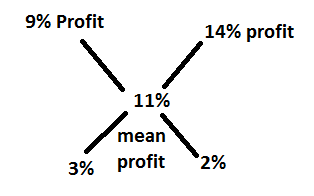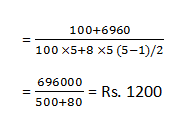# Numerical Ability Test for RBI Assistant Exam (Part: 2)1. After replacing an old member by a new member, it was found that the average age of eight members of a club is the same as it was 3 years ago.
What is the difference between the ages of the replaced and the new member?
1. 32 years
2. 20 years
3. 28 years
4. 22 years
5. 24 years

2. A trader has 65 kg of rice, a part of which he sells at 9% profit and the rest at 14% profit. He gains 11% on the whole. What is the quantity sold at 9% profit?
1. 28 kg
2. 39kg
3. 26 kg
4. 32 kg
5 36kg

3. What annual payment will discharge a debt of Rs. 6960 due in 5 years. if the rate of interest is 8% per annum?
1. Rs. 1200
2. Rs. 1400
3. Rs. 1600
4. Other than the given options
5. Rs. 1250

4. A dealer sold a washing machine at a loss of 3.5%. Had he sold it for Rs. 2054 more, he would have gained 9.5%.
For what value should sell it in order to gain 13 1/2%?
1. Rs. 18433
2. Rs. 18943
3. Rs. 17933
4. Rs. 17633
5. Rs. 17863

5. Anuradha Reena can do a work in 48 and 42 days respectively. They began the work together, but Anuradha left after some days and Reena finished the remaining work in 19 1/2 days. After how many days did Anuradha leave the work?
1. 15 days
2. 8 days
3. 16 days
4. 10 days
5. 12 days

6. Rohan leaves point A and reaches point B in 6 hours. Anuj leaves point B I hour earlier than Rohan and reaches the point A in 8 hours. Find the time in which Rohan meets Anuj.
1. 4 1/3 days
2. 3 hours
3. 2 1/3 days
4. 4 1/2 hours
5. 3 1/3 hours

7. In the stream running at 2km/he a motor boat goes 14 km upstream and back to the starting point in 77 minutes. Find the speed of the motor boat in still water.
1. Other than the given option
2. 21km/hr
3. 24 km/hr
4. 22 km/hr
5. 20 km/hr

8. A room 57m 80cm long and 47m 60cm broad is to be paved with equal square tiles. Find the largest tile so that the exactly fit and also find the number of tiles required.
1. 3.8m, 224
2. 3.6m, 264
3. 3.4m, 243
4. 3.4m, 240
5. 3.4m, 238

9. When two dice are thrown, what is the probability that the sum of the numbers is a multiple of four?
1. 1/4
2. 1/2
3. Other than the given options
4. 3/4
5. 2/9

1.
Age decreased = 8 x 3 = 24 years
So, the required difference = 24 years

2.
By Alligation Method:∴ Required ratio= 3:2
∴ Quantity sold at 9% profit = 3/3+2 x 65 = 39 kg

3. 1;
Annual payment4. 3;
Let the cost price Rs. X5. 5;
Let Anuradha left the work after x days6. 2;
Required time7. 4;
Let the speed of the motor boat in still water be x km/hr.8. 5;
Largest possible tile = HCF of length and breadth of the room = 3.4 m.
Number of tiles required9. 1;
Favourable event, = {(1,3); (2,2); (2,6), (3,1); (3,5), (4,4); (5,3); (6,2); (6,6)} =9
∴ Required probability = 9/36 = 1/4